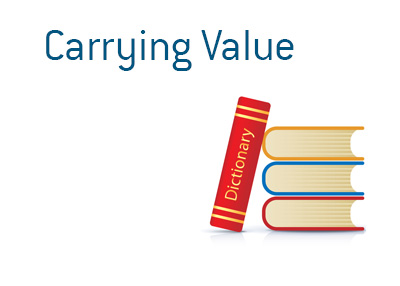## Definition of Carrying Value

What does the term "carrying value" mean in the world of finance? What is the definition of the term "carrying value"?

In finance, the term "carrying value" is used to show the remaining value of an asset on a company's books.In order to calculate "carrying value", this calculation must be used:

Purchase Price - Depreciation - Impairments = Carrying Value

So, if an asset was purchased for \$100,000 and had recorded depreciation of \$25,000 and impairment charges of \$15,000, the carrying value of the assets on the company's books would be:

\$100,000 (Purchase Price) - \$25,000 (Depreciation) - \$15,000 (Asset Impairment) = \$60,000

So, the "carrying value" of this asset would be \$60,000.

It should be noted that "carrying value" is not to be confused with "market value", which is the value that an asset could fetch on the open market. Carrying value and market value are two different things - an asset that has a carrying value of \$60,000 on a company's books may only fetch \$45,000 in the marketplace.

--

Davemanuel.com Articles That Mention Carrying Value:

None